# CUDA C/C++ 教程一：加速应用程序### 1. CUDA 基础

#### 1.1. CUDA 简介

GPU 加速计算正在逐步取代 CPU 计算，近年来加速计算带来了越来越多的突破性进展，各类应用程序对加速计算日益增长地需求、便捷地编写加速计算的程序的需求以及不断改进的支持加速计算的硬件设施，所有这一切都在推动着计算方式从 CPU 计算过渡到 GPU 加速计算。

CUDA 支持许多领域的超性能计算应用程序：计算流体动力学、分子动力学、量子化学、物理学 和高性能计算 (HPC)等等。

#### 1.2. 学习前的准备工作

• 在 C++/C 中声明变量、编写循环并使用 if/else 语句。
• 在 C++/C 中定义和调用函数。
• 在 C++/C 中分配数组。

• 编写、编译及运行既可调用 CPU 函数可启动 GPU 核函数的 C/C++ 程序。
• 通过配置参数控制并行线程的层次结构。
• 重构串行循环以在 GPU 上并行执行其迭代。
• 分配和释放可用于 CPU 和 GPU 的内存。
• 处理 CUDA 代码产生的错误。
• 加速 CPU 应用程序。

#### 1.3. 加速系统的硬件设施

nvidia-smi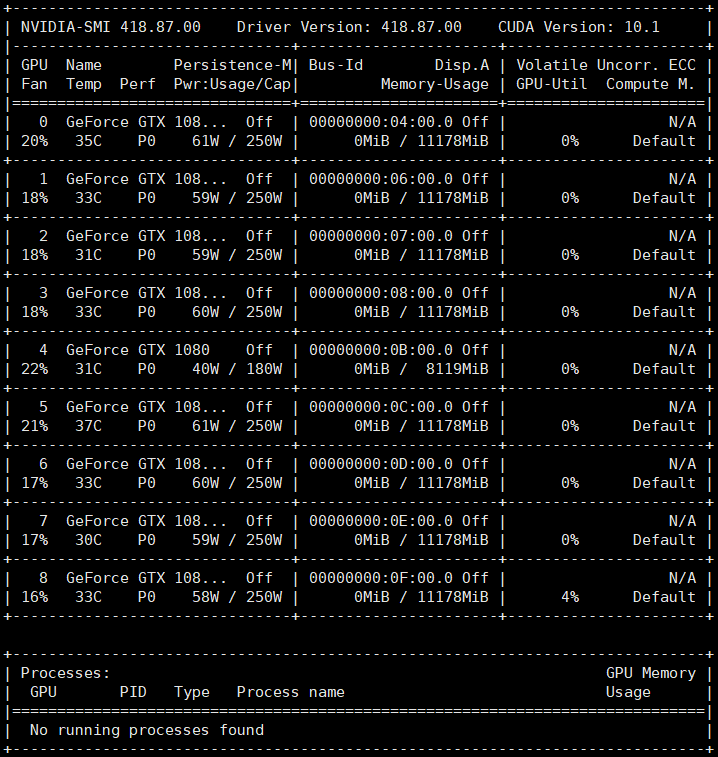### 2. 编写在GPU运行的代码

CUDA 为许多编程语言提供了扩展接口，而在本教程用CUDA为 C/C++ 提供的接口来展示。对编程语言的扩展可以让开发人员在 GPU 上更加方便的运行 CUDA 库的函数。

// 在CPU上运行的函数
void CPUFunction() {
printf("This function is defined to run on the CPU.\n");
}

// 在GPU上运行的函数
__global__ void GPUFunction() {
printf("This function is defined to run on the GPU.\n");
}

int main() {
CPUFunction(); // 调用CPU函数

GPUFunction<<<1, 1>>>(); // 调用GPU函数
}


__global__ void GPUFunction()

• __global__ 关键字表明该函数将在 GPU 上运行并可全局调用（ 既可以由CPU ，也可以由 GPU 调用）；
• 通常，我们将在 CPU 上执行的代码称为 Host （主机）代码，而将在 GPU 上运行的代码称为 Device （设备）代码；
• 注意返回类型为 void。使用 __global__ 关键字定义的函数返回值需为 void 类型。

GPUFunction<<<1, 1>>>()

• 通常，我们把要运行在 GPU 上的函数称为 kernel （核）函数;
• 启动核(kernel)函数时，我们必须事先配置GPU参数，使用 <<< ... >>> 语法向核函数传递两个必要的参数;
• <<< ... >>> 中传递的参数用于为核函数设定线程的层次结构，第一个参数定义线程块(Block)的数量，第二个参数定义Block中含有的线程(Thread)数量。例如本例中的核函数 GPUFunction() 将在包含 1 个线程（第二个配置参数）的 1 个线程块（第一个执行配置参数）上运行。

cudaDeviceSynchronize()

• 与其他并行化的代码类似，核函数启动方式为异步，即 CPU 代码将继续执行而不会等待核函数执行完成；
• 调用 CUDA 提供的函数 cudaDeviceSynchronize 可以让Host 代码(CPU) 等待 Device 代码(GPU) 执行完毕，再在CPU上继续执行。

#### 2.1. 编写运行一个 Hello GPU 核函数

#include <stdio.h>

void helloCPU() {
printf("Hello from the CPU.\n");
}

// __global__ 表明这是一个全局GPU核函数.
__global__ void helloGPU() {
printf("Hello from the GPU.\n");
}

int main() {
helloCPU(); // 调用CPU函数

/* 使用 <<<...>>> 配置核函数的GPU参数，
* 第一个1表示1个线程块，第二个1表示每个线程块1个线程。*/
helloGPU<<<1, 1>>>(); // 调用GPU函数
cudaDeviceSynchronize(); // cudaDeviceSynchronize 同步CPU和GPU
}


nvcc hello-gpu.cu -o hello-gpu
./hello-gpu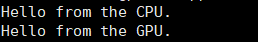### 3. CUDA 线程的层次结构#### 3.1. 运行核函数

<<< Block 数, 每个Block中的 Thread 数>>>


• someKernel<<<1, 1>>() 在GPU中为该核函数分配1个具有1个线程的线程块，核函数中的代码将只运行1次；
• someKernel<<<1, 10>>() 在GPU中为该核函数分配1个具有10个线程的线程块，核函数中的代码将运行10次；
• someKernel<<<10, 1>>() 在GPU中为该核函数分配10个具有1个线程的线程块，核函数中的代码将运行10次；
• someKernel<<<10, 10>>() 在GPU中为该核函数分配10个具有10个线程的线程块，核函数中的代码将运行100次；

#include <stdio.h>

__global__ void firstParallel() {
printf("This is running in parallel.\n");
}

int main() {
firstParallel<<<5, 5>>>(); // 在GPU中为核函数分配5个具有5个线程的线程块，将运行25次；
}


nvcc basic-parallel.cu -o basic-parallel
./basic-parallel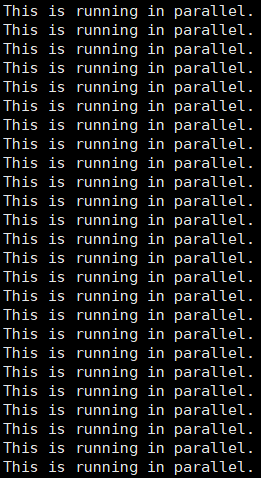#### 3.2. 线程和块的索引#include <stdio.h>

// 核函数
__global__ void printSuccessForCorrectExecutionConfiguration() {
// 当执行到第255个线程块的第1023个线程时，才输出
if(threadIdx.x == 1023 && blockIdx.x == 255) {
printf("Success!\n"); // 输出 Success！
printf("blockIdx.x: %d\n", blockIdx.x); // 输出线程块ID
}
}

int main() {
// 配置该核函数由256个含有1024个线程的线程块中执行
printSuccessForCorrectExecutionConfiguration<<<256, 1024>>>();
}


nvcc thread-and-block-idx.cu -o thread-and-block-idx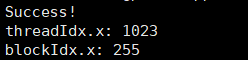#### 3.3. 用 CUDA 加速 For 循环

int N = 10;
for (int i = 0; i < N; ++i) {
printf("%d\n", i);
}


• 编写用于执行单次迭代工作的核函数。
• 调用核函数时为它配置执行参数，即并行的线程数，每个线程执行一次迭代。

#include <stdio.h>

// 核函数
__global__ void loop() {
// 输出每一个线程的线程号（0~9）
printf("This is iteration number %d\n", threadIdx.x);
}

int main() {
loop<<<1, 10>>>(); // 执行核函数
}


nvcc single-block-loop.cu -o single-block-loop
./single-block-loop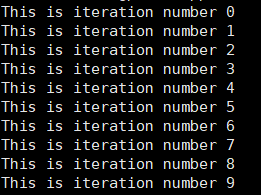#### 3.4. 管理不同块之间的线程

CUDA 核函数中，记录了每个块中线程数的变量是 blockDim.x（一个线程块中包含的线程数量，每个块中包含的线程数都是一样的）。通过将此变量与 blockIdx.xthreadIdx.x 变量结合使用，并借助表达式 threadIdx.x + blockIdx.x * blockDim.x 计算线程ID。该表达式可以用C++中访问二维数组的索引计算来类比看待，以增强理解。

• 如果线程块 blockIdx.x 索引为 0，则 blockIdx.x * blockDim.x 为 0。以 0 为起始索引加上可能的 threadIdx.x 值（0 至 9），便可在网格中找到索引为 0 至 9 的线程。
• 如果线程块 blockIdx.x 索引为 1，则 blockIdx.x * blockDim.x 为 10。以 10 为起始索引加上可能的 threadIdx.x 值（0 至 9），便可在网格中找到索引为 10 至 19 的线程。
• 如果线程块 blockIdx.x 索引为 5，则 blockIdx.x * blockDim.x 为 50。以 50 为起始索引加上可能的 threadIdx.x 值（0 至 9），便可在网格中找到索引为 50 至 59 的线程。
• 如果线程块 blockIdx.x 索引为 9，则 blockIdx.x * blockDim.x 为 90。以 90 为起始索引加上可能的 threadIdx.x 值（0 至 9），便可在网格中找到索引为 90 至 99 的线程。

#include <stdio.h>

__global__ void loop()
{
int i = blockIdx.x * blockDim.x + threadIdx.x;
printf("%d\n", i);
}

int main()
{
/*
* 配置参数还可以试试其他的，例如：
* <<<5, 2>>>
* <<<10, 1>>>
*/
loop<<<2, 5>>>();
}


nvcc multi-block-loop.cu -o multi-block-loop
./multi-block-loop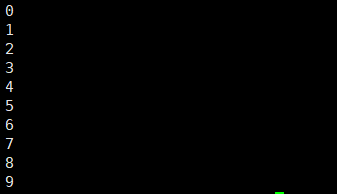### 4. 分配可同时被GPU和CPU访问的内存

CUDA 的最新版本（版本 6 和更高版本）可以便捷地分配和释放既可用于 Host 也可被 Device 访问的内存。

// CPU
int N = 10;
size_t size = N * sizeof(int);
int *a;

a = (int *)malloc(size); // 分配CPU内存
free(a); // 释放CPU内存

// GPU
int N = 10;
size_t size = N * sizeof(int);
int *a;

cudaMallocManaged(&a, size);// 为a分配CPU和GPU内存
cudaFree(a); // 释放GPU内存


#include <stdio.h>

// 初始化数组
void init(int *a, int N) {
int i;
for (i = 0; i < N; ++i) {
a[i] = i;
}
}

// CUDA 核函数，所有元素乘2
__global__ void doubleElements(int *a, int N) {
int i;
i = blockIdx.x * blockDim.x + threadIdx.x;
if (i < N) {
a[i] *= 2;
}
}

// 检查数组内所有元素的值是否均为复数
bool checkElementsAreDoubled(int *a, int N) {
int i;
for (i = 0; i < N; ++i) {
if (a[i] != i*2) return false;
}
return true;
}

int main() {
int N = 1000;
int *a;

size_t size = N * sizeof(int);
cudaMallocManaged(&a, size); // 为a分配CPU和GPU空间

init(a, N); // 为数组a赋值
size_t number_of_blocks = (N + threads_per_block - 1) / threads_per_block; // 定义block的数量

bool areDoubled = checkElementsAreDoubled(a, N); // 检查元素是否为复数
printf("All elements were doubled? %s\n", areDoubled ? "TRUE" : "FALSE");

cudaFree(a); // 释放由cudaMallocManaged
}


nvcc double-elements.cu -o double-elements
./double-elements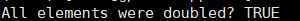### 5. 网格大小与实际并行工作量不匹配#### 5.1. 网格大于工作量

• 首先，设置配置参数，使线程总数超过实际工作所需的线程数。
• 然后，在向核函数传递参数时传递一个用于表示要处理的数据集总大小或完成工作所需的总线程数 N。
• 最后，计算网格内的线程索引后（使用 threadIdx + blockIdx*blockDim），判断该索引是否超过 N，只在不超过的情况下执行与核函数相关的工作。

// 假设N是已知的
int N = 100000;



__global__ some_kernel(int N) {
int idx = threadIdx.x + blockIdx.x * blockDim.x;

if (idx < N) { // 保证线程ID小于元素数量N
// 并行代码
}


#include <stdio.h>

__global__ void initializeElementsTo(int initialValue, int *a, int N) {
int i = threadIdx.x + blockIdx.x * blockDim.x;
if (i < N) {
a[i] = initialValue;
}
}

int main() {
int N = 1000;

int *a;
size_t size = N * sizeof(int);
cudaMallocManaged(&a, size);

// 这是惯用的CUDA语法
// 为 number_of_blocks 分配一个值，以确保线程数至少与指针 a 中可供访问的元素数同样多。

int initialValue = 6; // 初始化的值

// 检查元素值是否被初始化
for (int i = 0; i < N; ++i) {
if(a[i] != initialValue) {
printf("FAILURE: target value: %d\t a[%d]: %d\n", initialValue, i, a[i]);
exit(1);
}
}
printf("SUCCESS!\n");

cudaFree(a);
}


nvcc mismatched-config-loop.cu -o mismatched-config-loop
./mismatched-config-loop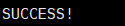#### 5.2. 网格小于工作量• a 执行相应运算；
• 将线程索引增加 250，使网格的大小达到 270
• a 执行相应运算；
• 将线程索引增加 250，使网格的大小达到 520
• 由于 520 现已超出数组范围，因此线程将停止工作。

CUDA 提供一个记录了网格中线程块数的变量：gridDim.x。然后可以利用它来计算网格中的总线程数，即网格中的线程块数乘以每个线程块中的线程数：gridDim.x * blockDim.x。现在来看看以下核函数中网格跨度循环的示例：

__global void kernel(int *a, int N)
{
int indexWithinTheGrid = threadIdx.x + blockIdx.x * blockDim.x;
int gridStride = gridDim.x * blockDim.x; // grid 的一个跨步

for (int i = indexWithinTheGrid; i < N; i += gridStride) {
// 对 a[i] 的操作;
}
}


#include <stdio.h>

// 初始化数组a
void init(int *a, int N) {
int i;
for (i = 0; i < N; ++i) {
a[i] = i;
}
}

__global__ void doubleElements(int *a, int N) {

// 使用grid-stride循环，这样每个线程可以处理数组中的多个元素。
int idx = blockIdx.x * blockDim.x + threadIdx.x;
int stride = gridDim.x * blockDim.x; // grid 的一个跨步

for (int i = idx; i < N; i += stride) {
a[i] *= 2;
}
}

// 检查数组内所有元素的值是否均为复数
bool checkElementsAreDoubled(int *a, int N) {
int i;
for (i = 0; i < N; ++i) {
if (a[i] != i*2) return false;
}
return true;
}

int main() {
int N = 10000;
int *a;
size_t size = N * sizeof(int);
cudaMallocManaged(&a, size);

init(a, N); // 初始化数组a

size_t number_of_blocks = 32; // block数量

bool areDoubled = checkElementsAreDoubled(a, N);
// 检查数组内所有元素的值是否均为复数
printf("All elements were doubled? %s\n", areDoubled ? "TRUE" : "FALSE");

cudaFree(a);
}


nvcc grid-stride-double.cu -o grid-stride-double
./grid-stride-double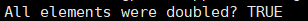### 6. 错误处理

CUDA 函数发生错误时会返回一个类型为 cudaError_t 的变量，该变量可用于检查调用函数时是否发生错误。以下是对调用 cudaMallocManaged 函数执行错误处理的示例：

cudaError_t err;
err = cudaMallocManaged(&a, N)                    // 假设a和N已经被定义

if (err != cudaSuccess) { // cudaSuccess 是一个 CUDA 变量.
printf("Error: %s\n", cudaGetErrorString(err)); // cudaGetErrorString 是一个 CUDA 函数.
}


// 这段程序中的核函数会出一个CUDA错误，但是核函数本身无法捕获该错误
someKernel<<<1, -1>>>();  // 线程数不能为-1

cudaError_t err;
err = cudaGetLastError(); // cudaGetLastError 会捕获上面代码中的最近的一个错误
if (err != cudaSuccess) {
printf("Error: %s\n", cudaGetErrorString(err));
}


#include <stdio.h>

// 初始化数组a
void init(int *a, int N) {
int i;
for (i = 0; i < N; ++i) {
a[i] = i;
}
}

// CUDA 核函数 数组元素值乘2
__global__ void doubleElements(int *a, int N) {
int idx = blockIdx.x * blockDim.x + threadIdx.x;
int stride = gridDim.x * blockDim.x;

// for (int i = idx; i < N; i += stride) {
// 这里出现一个数值越界错误
for (int i = idx; i < N + stride; i += stride) {
a[i] *= 2;
}
}

// 检查数组元素是否均为复数
bool checkElementsAreDoubled(int *a, int N) {
int i;
for (i = 0; i < N; ++i) {
if (a[i] != i*2) return false;
}
return true;
}

int main() {
int N = 10000;
int *a;

size_t size = N * sizeof(int);
cudaMallocManaged(&a, size);
init(a, N);

cudaError_t syncErr, asyncErr; // 定义错误处理变量

// 线程数大于1024（前面说过每个block的线程数不能超过1024）
size_t number_of_blocks = 32;

syncErr = cudaGetLastError(); // 捕获核函数执行期间发生的错误

// 输出错误 说明：两个错误需分别设置（即每次运行时只保留一个错误）
if (syncErr != cudaSuccess) printf("Error: %s\n", cudaGetErrorString(syncErr));
if (asyncErr != cudaSuccess) printf("Error: %s\n", cudaGetErrorString(asyncErr));

bool areDoubled = checkElementsAreDoubled(a, N); // 验证数组元素值是否均为复数
printf("All elements were doubled? %s\n", areDoubled ? "TRUE" : "FALSE");

cudaFree(a);
}


nvcc add-error-handling.cu -o add-error-handling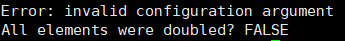#### 6.1. 定制一个 CUDA 错误处理宏

#include <stdio.h>
#include <assert.h>

// CUDA 错误处理宏
inline cudaError_t checkCuda(cudaError_t result)
{
if (result != cudaSuccess) {
fprintf(stderr, "CUDA Runtime Error: %s\n", cudaGetErrorString(result));
assert(result == cudaSuccess);
}
return result;
}

int main() {
// checkCuda 宏可以返回 CUDA 函数返回的错误类型cudaError_t的值
}


### 7. 总结

• 编写、编译及运行既可调用 CPU 函数也可启动GPU核函数的 C/C++ 程序。
• 使用执行配置控制并行线程层次结构。
• 重构串行循环以在 GPU 上并行执行其迭代。
• 分配和释放可用于 CPU 和 GPU 的内存。
• 处理 CUDA 代码生成的错误。

#### 7.1 用 CUDA 实现向量加法

#include <stdio.h>
#include <assert.h>

// CUDA 错误处理宏
inline cudaError_t checkCuda(cudaError_t result)
{
if (result != cudaSuccess) {
fprintf(stderr, "CUDA Runtime Error: %s\n", cudaGetErrorString(result));
assert(result == cudaSuccess);
}
return result;
}

// 初始化数组 a
void initWith(float num, float *a, int N) {
for(int i = 0; i < N; ++i) {
a[i] = num;
}
}

// 向量加法核函数
__global__ void addVectorsInto(float *result, float *a, float *b, int N) {
int index = threadIdx.x + blockIdx.x * blockDim.x;
int stride = blockDim.x * gridDim.x;

for(int i = index; i < N; i += stride) {
result[i] = a[i] + b[i]; // 元素a[i] + 元素 b[i]
}
}

// 检查 CUDA 向量加分是否计算正确
void checkElementsAre(float target, float *array, int N) {
for(int i = 0; i < N; i++) {
if(array[i] != target) {
printf("FAIL: array[%d] - %0.0f does not equal %0.0f\n", i, array[i], target);
exit(1);
}
}
}

int main() {
const int N = 10;
size_t size = N * sizeof(float);

float *a;
float *b;
float *c;

// 分配内存，且检查执行期间发生的错误
checkCuda( cudaMallocManaged(&a, size) );
checkCuda( cudaMallocManaged(&b, size) );
checkCuda( cudaMallocManaged(&c, size) );

initWith(3, a, N); // 将数组a中所有的元素初始化为3
initWith(4, b, N); // 将数组b中所有的元素初始化为4
initWith(0, c, N); // 将数组c中所有的元素初始化为0，数组c是结果向量

// 配置参数

checkCuda( cudaGetLastError() ); // 检查核函数执行期间发生的错误

checkElementsAre(7, c, N);  // 检查向量加的结果是否正确

// 释放内存，且检查执行期间发生的错误
checkCuda( cudaFree(a) );
checkCuda( cudaFree(b) );
checkCuda( cudaFree(c) );
}



#### 7.2. 二维和三维的网格和块

dim3 threads_per_block(16, 16, 1);
dim3 number_of_blocks(16, 16, 1);


#### 7.3 用 CUDA 实现矩阵乘法

#include <stdio.h>
#define N  64

// GPU 矩阵乘法
__global__ void matrixMulGPU( int * a, int * b, int * c ) {
int val = 0;

int row = blockIdx.x * blockDim.x + threadIdx.x;
int col = blockIdx.y * blockDim.y + threadIdx.y;

if (row < N && col < N) {
for ( int k = 0; k < N; ++k )
val += a[row * N + k] * b[k * N + col];
c[row * N + col] = val;
}
}

// CPU矩阵乘法
void matrixMulCPU( int * a, int * b, int * c ) {
int val = 0;

for( int row = 0; row < N; ++row )
for( int col = 0; col < N; ++col ) {
val = 0;
for ( int k = 0; k < N; ++k )
val += a[row * N + k] * b[k * N + col];
c[row * N + col] = val;
}
}

int main() {
int *a, *b, *c_cpu, *c_gpu;
int size = N * N * sizeof (int); // Number of bytes of an N x N matrix

// 分配内存
cudaMallocManaged (&a, size);
cudaMallocManaged (&b, size);
cudaMallocManaged (&c_cpu, size);
cudaMallocManaged (&c_gpu, size);

// 初始化数组
for( int row = 0; row < N; ++row )
for( int col = 0; col < N; ++col )
{
a[row * N + col] = row;
b[row * N + col] = col + 2;
c_cpu[row * N + col] = 0;
c_gpu[row * N + col] = 0;
}

dim3 threads_per_block (16, 16, 1); // 一个 16 * 16 的线程阵
dim3 number_of_blocks ((N / threads_per_block.x) + 1, (N / threads_per_block.y) + 1, 1);
matrixMulGPU <<< number_of_blocks, threads_per_block >>> ( a, b, c_gpu ); // 执行核函数

matrixMulCPU( a, b, c_cpu ); // 执行 CPU 版本的矩阵乘法

// 比较 CPU 和 GPU 两种方法的计算结果是否一致
bool error = false;
for( int row = 0; row < N && !error; ++row )
for( int col = 0; col < N && !error; ++col )
if (c_cpu[row * N + col] != c_gpu[row * N + col]) {
printf("FOUND ERROR at c[%d][%d]\n", row, col);
error = true;
break;
}
if (!error)
printf("Success!\n");

// 释放内存
cudaFree(a); cudaFree(b);
cudaFree( c_cpu ); cudaFree( c_gpu );
}

02-145695
11-06

05-18115
05-2088
05-241336
10-202544
02-23747
05-202814
06-119162
02-23786
04-233123
05-041142
09-251015
11-161658
12-19307
06-291万+
06-04359
10-092134
12-035532
12-16581

### “相关推荐”对你有帮助么？

•非常没帮助
•没帮助
•一般
•有帮助
•非常有帮助¥2 ¥4 ¥6 ¥10 ¥20余额支付 (余额：-- )扫码支付获取中扫码支付点击重新获取扫码支付1.余额是钱包充值的虚拟货币，按照1:1的比例进行支付金额的抵扣。
2.余额无法直接购买下载，可以购买VIP、C币套餐、付费专栏及课程。余额充值